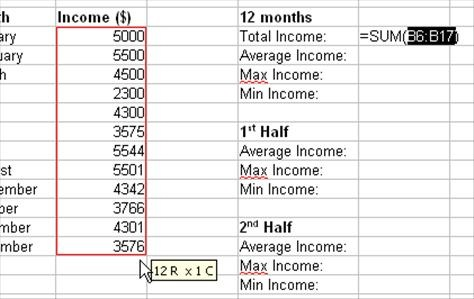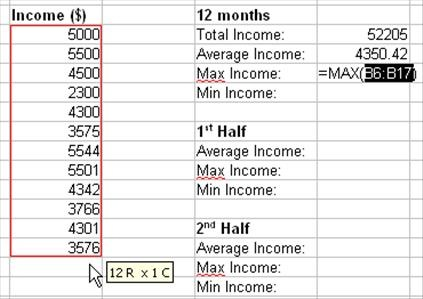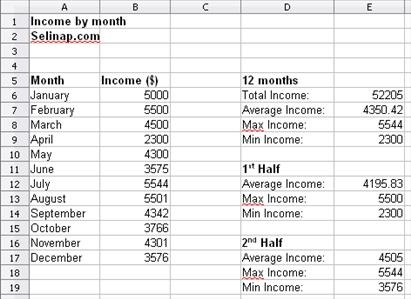## Friday, January 9, 2009

This tutorial is about creating a simple spreadsheet to monitor monthly income. The spreadsheet will contains income by month, and total, average, max, and min income for the whole year. Besides, the spreadsheet will also provides the average, max and min income for the 1st and second half of the year.

Here are the steps:
1. Calculate total income.
Click on cell E6. Type "=SUM(" without the quotes. Do not close the bracket yet. Select cells B6 to B17. Close the bracket. Press Enter.2. Calculate average income.
Repeat the same procedure as in step 1, but now use "AVERAGE" instead of "SUM".3. Calculate max income.
Repeat the same procedure as in step 1, but now use "MAX" instead of "SUM".4. Calculate min income.
Repeat the same procedure as in step 1, but now use "MIN" instead of "SUM".5. Calculate average income for the 1st half of the year.
Repeat the same procedure as in step 1, but only select 6 rows of cells (B6 to B11), since we need to calculate the average of income for 6 months.6. Calculate max income for the 1st half of the year.7. Calculate min income for the 1st half of the year.8. Repeat step 5, 6 and 7 for average, max and min income for the 2nd half of the year, by selecting the rows for month July to December.

The final product should more or less, look like the picture below.Please note that the spreadsheet used in this tutorial is OpenOffice Calc. However, the same formulas will also work in Microsoft Excel.

1.ohh what a nice way to monitor it.~

<abbr>harmonys last blog post..Friday's Get it over and done with Today.</abbr>

2.thanks for the tips
aku jarang buat monitoring camni. hehe :)

3.aku pun. jarang pula buat camni. thanks for the info.

<abbr>alones last blog post..Save Gaza - Save our Brothers & Sisters</abbr>